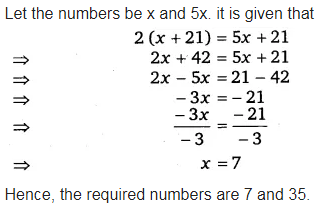# A positive number is 5 times another number. If 21 is added to both the numbers,

A positive number is 5 times another number. If 21 is added to both the numbers, then one of the new numbers becomes twice the other new number. What are the numbers ?